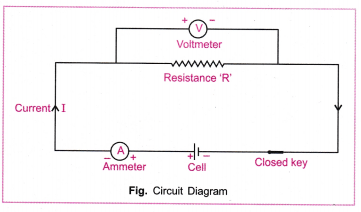# State Ohm's law. Give Mathematical relation between potential difference (V), Current (I) and resistance (R) of a conductor. Draw an electric circuit for studying ohm's law.

Ohm's law :

Ohm's law states that at a constant temperature, the current passing through a conductor is directly proportional to the potential difference applied across it.

So, potential difference V is directly proportional to the electric current I.
Or, $V \propto I$
$\mathrm{Or}, \frac{V}{I}=Constant$
This constant is called resistance R. Resistance is the property of conductor which resists the flow of electric current through it.
Therefore, $\frac{V}{I}=R$
$\mathrm{Or}, \frac{1}{I}=\frac{R}{V}$
$\mathrm{Or}, I=\frac{V}{R}$
$\mathrm{Or}, V=R I$
The following electric circuit is used for studying Ohm's law.